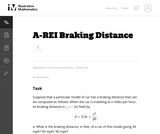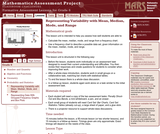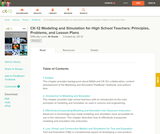# 3 Results

View
Selected filters:
• ModelingUnrestricted Use
CC BY
Rating

This task provides an exploration of a quadratic equation by descriptive, numerical, graphical, and algebraic techniques. Based on its real-world applicability, teachers could use the task as a way to introduce and motivate algebraic techniques like completing the square, en route to a derivation of the quadratic formula.

Material Type:
Activity/Lab
Provider:
Illustrative Mathematics
Provider Set:
Illustrative Mathematics
Author:
Illustrative Mathematics
05/01/2012Only Sharing Permitted
CC BY-NC-ND
Rating

This lesson unit is intended to help you assess how well students are able to: Calculate the mean, median, mode, and range from a frequency chart; and to use a frequency chart to describe a possible data set, given information on the mean, median, mode, and range.

Material Type:
Activity/Lab
Assessment
Lesson Plan
Provider:
Shell Center for Mathematical Education
U.C. Berkeley
Provider Set:
Mathematics Assessment Project (MAP)
Author:
Shell Center Team
01/17/2013Conditional Remix & Share Permitted
CC BY-NC-SA
Rating

A collaboration between the National Aeronautics and Space Administration (NASA) and the CK-12 Foundation, this book provides high school mathematics and physics teachers with an introduction to the main principles of modeling and simulation used in science and engineering. An appendix of lesson plans is included.

نوع المادة:
Lesson Plan
Teaching/Learning Strategy
Textbook
Provider:
CK-12 Foundation
Provider Set:
كتاب FlexBook من تصنيف المرحلة من الابتدائية إلى الثانوية